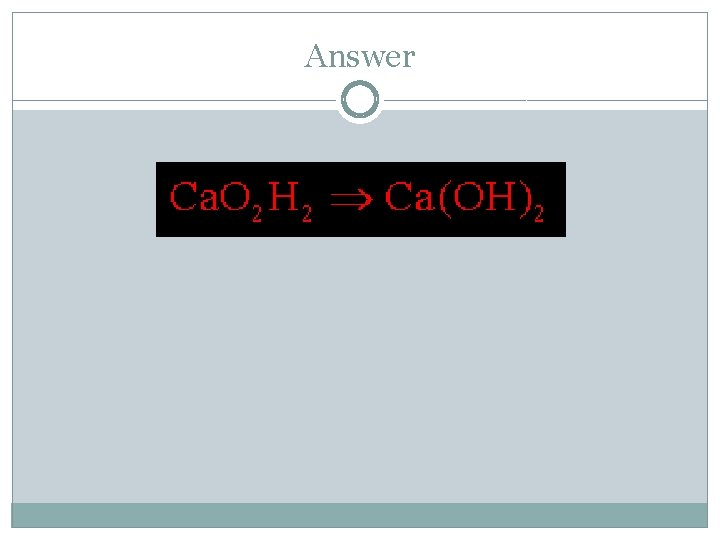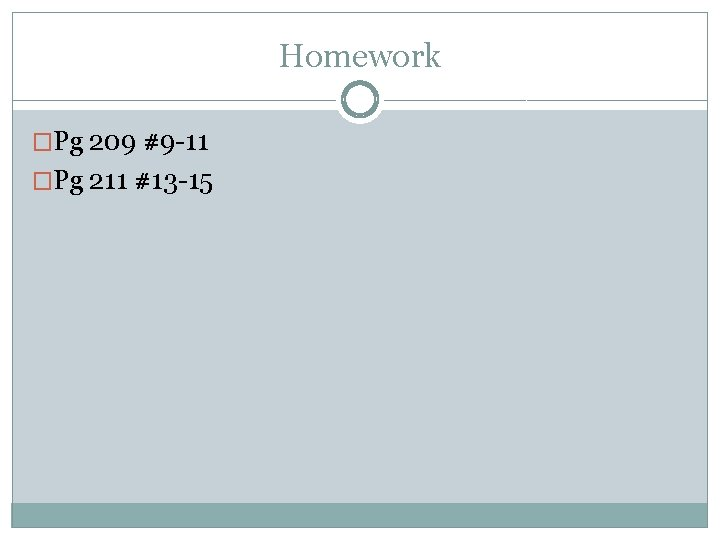# Emperical Formula Emperical Formula the empirical formula of

• Slides: 9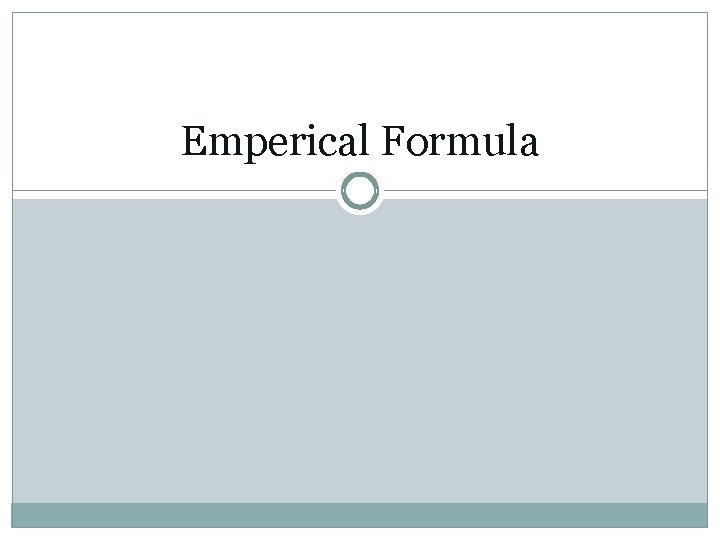Emperical Formula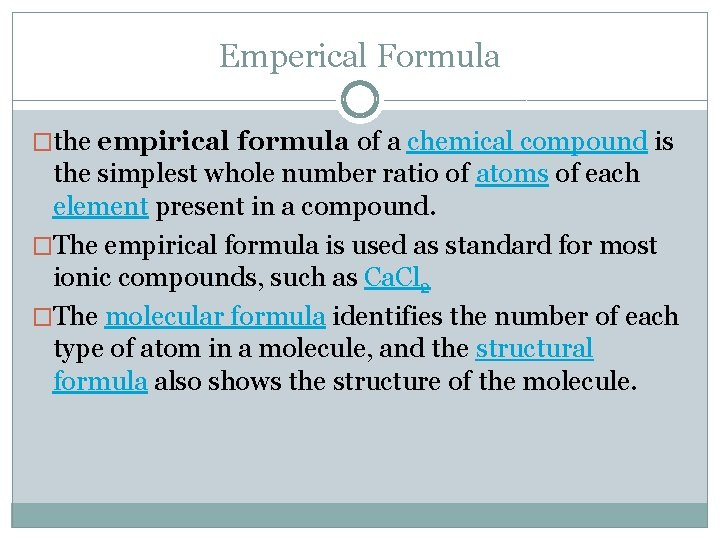Emperical Formula �the empirical formula of a chemical compound is the simplest whole number ratio of atoms of each element present in a compound. �The empirical formula is used as standard for most ionic compounds, such as Ca. Cl 2 �The molecular formula identifies the number of each type of atom in a molecule, and the structural formula also shows the structure of the molecule.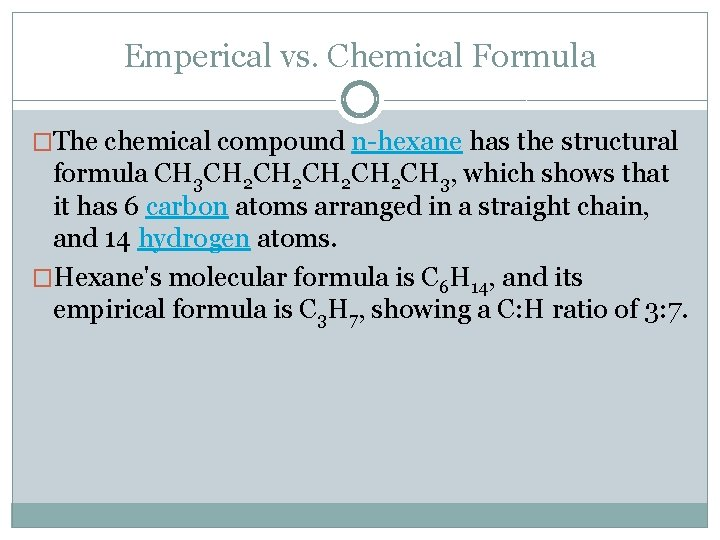Emperical vs. Chemical Formula �The chemical compound n-hexane has the structural formula CH 3 CH 2 CH 2 CH 3, which shows that it has 6 carbon atoms arranged in a straight chain, and 14 hydrogen atoms. �Hexane's molecular formula is C 6 H 14, and its empirical formula is C 3 H 7, showing a C: H ratio of 3: 7.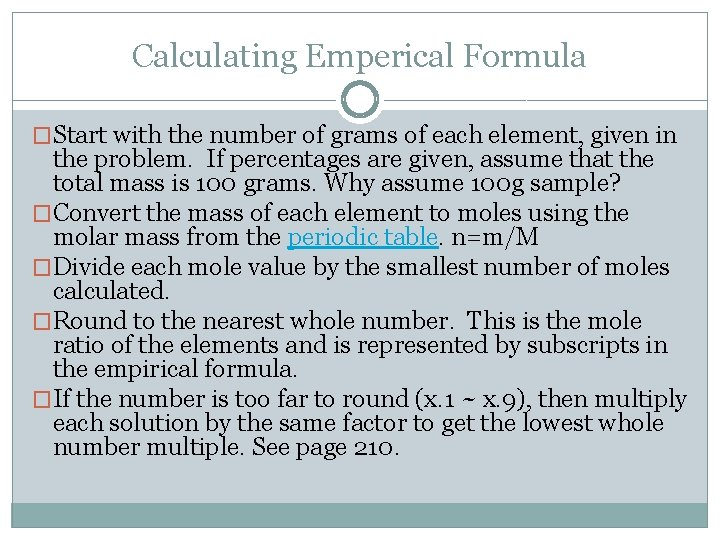Calculating Emperical Formula �Start with the number of grams of each element, given in the problem. If percentages are given, assume that the total mass is 100 grams. Why assume 100 g sample? �Convert the mass of each element to moles using the molar mass from the periodic table. n=m/M �Divide each mole value by the smallest number of moles calculated. �Round to the nearest whole number. This is the mole ratio of the elements and is represented by subscripts in the empirical formula. �If the number is too far to round (x. 1 ~ x. 9), then multiply each solution by the same factor to get the lowest whole number multiple. See page 210.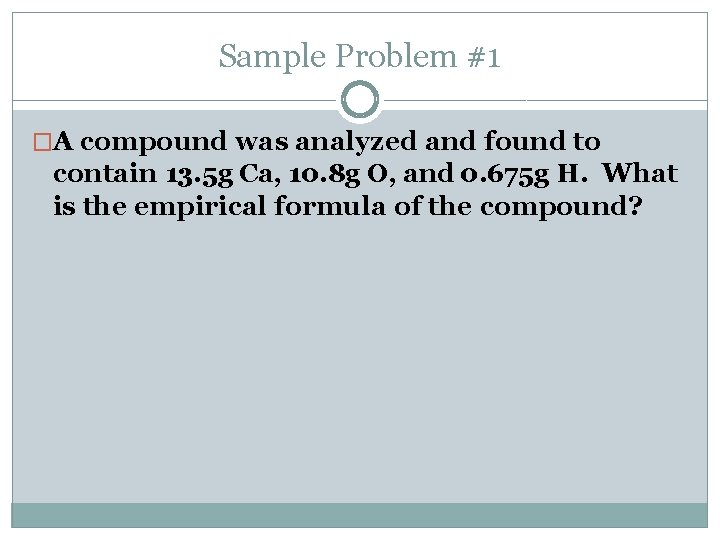Sample Problem #1 �A compound was analyzed and found to contain 13. 5 g Ca, 10. 8 g O, and 0. 675 g H. What is the empirical formula of the compound?